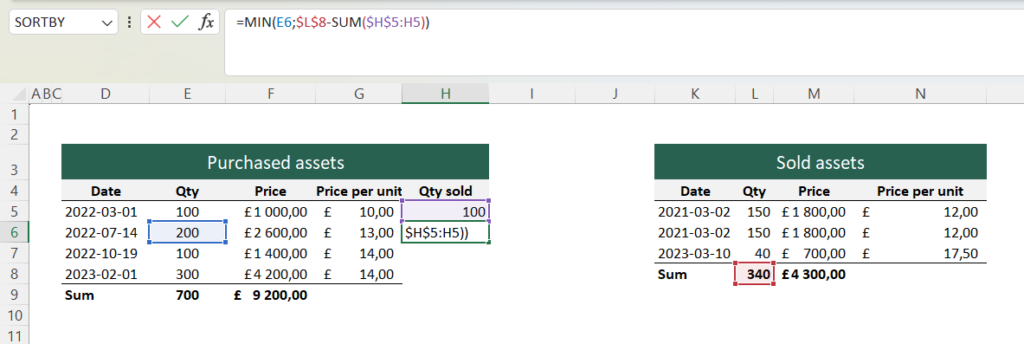# FIFO Calculation in Excel

The FIFO principle is an important principle in logistics, finance, and accounting. The abbreviation FIFO stands for ‘first in, first out,’ which means that assets purchased first should also be sold first.

Retrieve the data here: FIFO Calculation.xlsx

A FIFO calculation is required to calculate costs and profits for assets that:

1. Have been acquired at different prices in multiple rounds.
2. Have been sold at different prices in multiple rounds.
3. Cannot be uniquely identified.

In logistics, FIFO is applied to ensure that older goods are used or sold first, reducing the risk of selling goods that have expired or become obsolete.

In economic contexts, the principle is mostly applied to assets such as stocks, bonds, or currencies. When selling these assets, the capital gain or loss is often calculated by subtracting the cost basis from the selling price according to the FIFO principle.

To create a dynamic FIFO model in Excel:

1. Create two tables that store data on purchased and sold assets. Calculate the price per unit or the total price if either is missing.2. Then, calculate in a new column how many of the purchased assets have been sold. For the first purchase, the formula would be:
```=MIN( [quantity purchased in purchase no. 1] ; [quantity sold in total]```

The MIN function simply returns the smallest of its arguments and is used here to evaluate if all 100 units purchased on 01/03/2022 have already been sold.

1. For the next purchase, a similar formula applies, but now we need to consider the 100 units we have already sold. We then write a SUM formula that adds all the values above and subtracts them from the total number of units sold.We can now see how all 340 units sold have their own cost basis in the table for purchased assets.

1. Calculate the cost of goods sold by multiplying [Quantity sold] by [Price per unit].2. Finally, we obtain the profit/loss by subtracting the cost of goods sold from the revenues.The calculation is now complete and updates automatically when new rows are added to the tables. Note that this calculation only works for products of the same type. If you need multiple types, you can simply create separate sheets for each product.

Tip: You can further develop this model by, for example, calculating the inventory value of products that have not yet been sold!

### More blog postsTired of Googling?